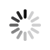#### Welcome!

×Forgot password?
Go back to Challenges list

Challenge

## Greatest Prime Tailored Number

In this challenge you will have to perform some operations with numbers.

You need to find the greatest prime divisor of a given number. However, before that, you need to check if the digits of a given number are ordered increasingly. If yes, the given number must be recalculated, and you should use as input the multiplication of all digits of that number. If not, the given number should remain untouchable.

NOTE:
If the number has two digits of the same value in a row can still count as ordered increasingly. (Example: 1223 is considered as ordered increasingly and will be equal to 1 x 2 x 2 x 3 = 12)

###### Input Format
Each test case contains a long number.

###### Output Format
For each test case output the greatest prime divisor of a tailored number.

`13`

`3`

`512M`